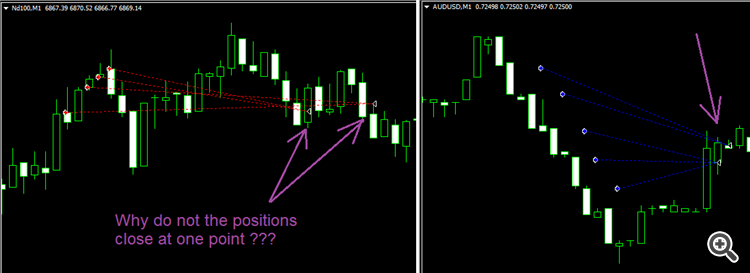# Difference at the exit point32

Hello
I have a problem running a programming code .When I'm reviewing my programming code in strategy tester (Every tick) and real market , I see the difference in the result. I don't know ,why I have two exit points.
Thanks```//Orders Counting & Close Orders------------------------------------+

int orderscnt=0;
for (int  i=OrdersTotal()-1 ; i>=0 ; i--)
{
//--------------------------------------
if((OrderSymbol()==Symbol()) && ((OrderType()==OP_SELL) || (OrderType()==OP_BUY))&& OrderMagicNumber()== MagicNumber)
{
orderscnt++;
}